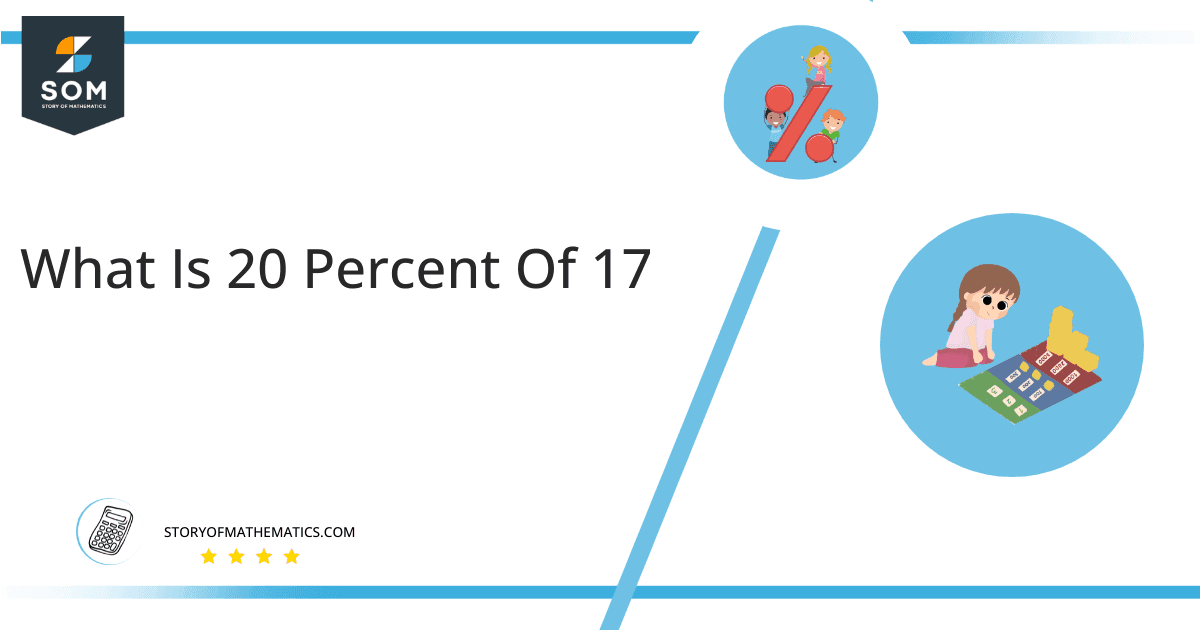# What Is 20 Percent of 17 + Solution with Free Steps?

The 20 percent of 17 is equal to 3.4. It can be easily calculated by dividing 20 by 100 and multiplying the answer with 17 to get 3.4.The easiest way to get this answer is by solving a simple mathematical problem of percentages. You need to find 20% of 17 for some sale or real-life problem. Divide 20 by 100, multiply the answer by 17, and get the 20% of 17 value in seconds.

This article will explain the full process of finding any percentage value from any given quantity or number with easy and simple steps.

## What Is 20 percent of 17?

The 20 percent of 17 is 3.4.

The percentage can be understood with a simple explanation. Take 17, and divide it into 100 equal parts. The 20 parts from the total of 100 parts is called 20 percent, which is 3.4 in this example.

## How To Calculate 20 percent of 17?

You can find 20 percent of 17 by some simple mathematical steps explained below.

### Step 1

Firstly, depict 20 percent of 17 as a fractional multiple as shown below:

20% x 17

### Step 2

The percentage sign % means percent, equivalent to the fraction of 1/100.

Substituting this value in the above formula:

= (20/100) x 17

### Step 3

Using the algebraic simplification process, we can arithmetically manipulate the above equation as follows:

= (20 x 17) / 100

= 340 / 100

= 3.4This percentage can be represented on a pie chart for visualization. Let us suppose that the whole pie chart represents the 17 values. Now, we find 20 percent of 17, which is 3.4. The area occupied by the 3.4 value will represent the 20 percent of the total 17 values. The remaining region of the pie chart will represent 80 percent of the total 17 values. The 100% of 17 will cover the whole pie chart as 17 is the total value.

Any given number or quantity can be represented in percentages to better understand the total quantity. The percentage can be considered a quantity that divides any number into hundred equal parts for better representation of large numbers and understanding.

Percentage scaling or normalization is a very simple and convenient method of representing numbers in relative terms. Such notations find wide application in many industrial sectors where the relative proportions are used.